# JavaScript Set Data Structure

## JavaScript Set Data Structure with Examples

In this article, I am going to discuss JavaScript Set Data Structure with Examples. Please read our previous article where we discussed the JavaScript Arrow function with Examples. JavaScript ECMAScript6 (ES6) introduces four new data structures: Set, WeakSet, Map, and WeakMap. Let’s start exploring and understanding in detail more about Set. At the end of this article, you will understand the following pointers in detail.

1. What is Set in JavaScript?
2. Where to use Set in JavaScript?
3. JavaScript Set Methods
4. How to Create a Set in JavaScript?
5. How to add value to a Set?
6. Removing a value from a set
7. Checking if a value exists in a Set
8. Clearing a Set in JavaScript
9. Getting Set Length
10. Converting Sets to arrays
11. Intersection and differences in Sets
12. Iterating Sets in JavaScript
13. Array Vs Set in JavaScript
##### What is Set in JavaScript?

The main Feature of Set is it doesn’t allow duplicate values-(unique values). ES6 provides a new data structure named Set that stores a collection of unique values of any type, whether primitive values (like strings or integers) or object references (like arrays or function).

A Set is a special type of collection – a “set of values” (without keys), where each value may occur only once. That is, it is a collection of unique values in the Set.

We can add items into a set and iterate them the same as we do in a JavaScript Array. Sets are like Arrays they store a sequence of values but unlike an array, we cannot add duplicates of the same value to a Set, meaning Set can store only unique values

##### Where to use Set in JavaScript?

This is useful in cases where we are faced with potential duplicates, but only want to store a single instance of that value. Any duplicates are ignored.

##### Features of Set
• Set – is a collection of unique values
• A Set object cannot contain duplicate values.
• A Set object iterates its elements in insertion order, meaning it will always return values in the order they were first added to the Set.

Syntax: The below syntax creates a new Set

new Set([iterable]); The Set constructor also accepts an optional iterable object.

iterable: If we provide an iterable object (usually as an array) to the Set constructor, all the elements of the iterable object will be added to the new set, in other words, copies values from it into the set. null is treated as undefined.

##### JavaScript Set Methods

The Set object provides the following useful methods: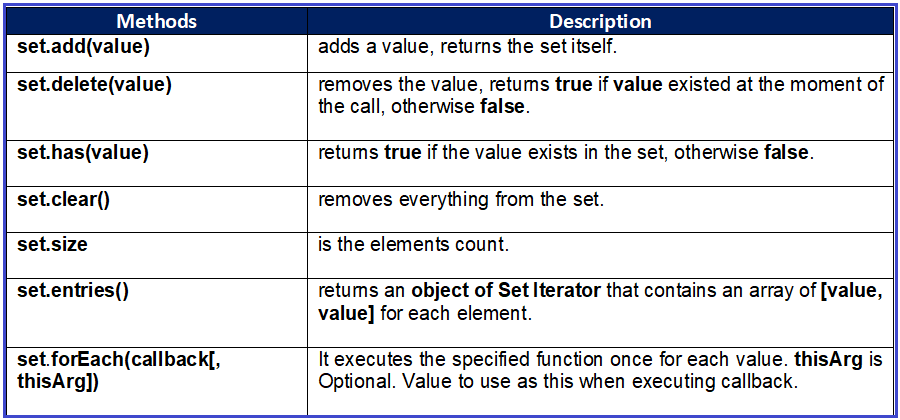##### Creating a Set in JavaScript

Creating a Set, like creating any object is extremely simple in JavaScript:
const mySet = new Set();

Or we can create a Set from any iterable object to give it starting values:
const arr = [1, 2, 3, 4, 4, 5];
const mySet = new Set(arr);

In the example above the Set, the content would be {1, 2, 3, 4, 5}. Note that the value 4 appears only once, unlike in the original array used to create it.

##### Example: JavaScript Set example
```<html>
<title>JavaScript Set example</title>
<body>
<script>
var mySet = new Set([1, 2, 2, 3, 4, 4]);
console.log("Set example:", mySet);
console.log("type of mySet:", typeof(mySet));
let result = mySet instanceof Set;
console.log("check if mySet instance of Set:", result);
</script>
</body>
</html>
```

Output: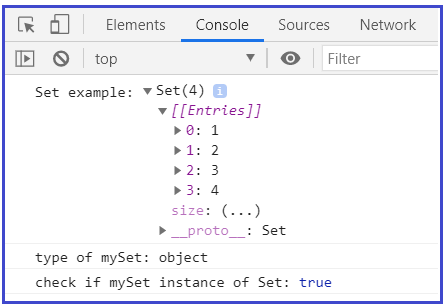All elements in the set must be unique therefore the mySet only contains 4 distinct elements 1,2,3, and 4. When we use typeof operator to the mySet, it returns an object. The mySet set is an instance of Set type hence the above result statement returns true.

##### Adding a value to a Set in JavaScript

Once we have created our Set, adding elements to it is similar to adding elements to an array. Simply call the add() method on the created Set with the element we want to add. To add value to a Set, use the add() method:

If the value already exists in the set it will not be added again, as Sets contain unique values. Note that the add() method returns the set itself, so we can chain add() calls together:

##### Example: JavaScript Set add() method example
```<html>
<body>
<script>
var mySet = new Set();
</script>
</body>
</html>
```

Output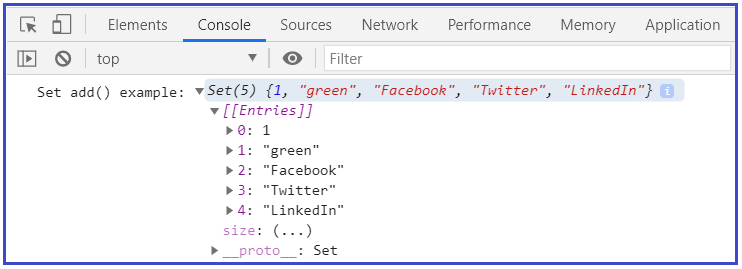##### Removing a value from a set

Deleting an element from a Set is as simple as calling the delete() method, and passing in the element we want to remove. To remove a value from a Set, use the delete() method:

mySet.delete(value); This function will return true if the value existed in the set and was removed, or false otherwise.

##### Example: JavaScript Set delete() method example
```<html>
<title>JavaScript Set delete() method example</title>
<body>
<script>
var mySet = new Set();

mySet.delete('Donut');
console.log("Set after Deleting value:", mySet);
</script>
</body>
</html>
```

Output: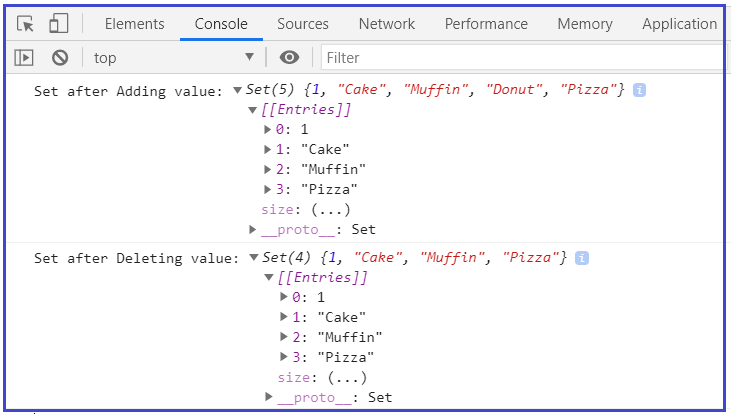##### Checking if a value exists in a Set

When we want to check if a Set contains an element, call the has() method on it. It returns a Boolean value(true/false) whether an element is present with the given value in the Set object or not. To check if a given value exists in a Set, use .has() method:

mySet.has(value); This function will return true if the value is present in the Set, or otherwise false.

##### Example: JavaScript Set has() method example
```<html>
<title>JavaScript Set has() method example</title>
<body>
<script>
var mySet = new Set();
console.log("Check if mySet contains Donut:", mySet.has('Donut'));
console.log("Check if mySet contains Pastry:", mySet.has('Pastry'));

var valueSet = new Set([1, 3, 7]);
console.log("Check if valueSet contains 3:", valueSet.has(3));
console.log("Check if valueSet contains 23:", valueSet.has(23));
</script>
</body>
</html>
```

Output: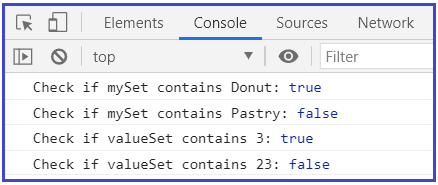##### Clearing a Set in JavaScript

To wipe a Set clean all of its elements, call the clear() method on it. It reset a Set as if we had just created a new one. We can remove all the elements in a Set using the clear() method:

mySet.clear();

##### Example: JavaScript Set clear() method example
```<html>
<title>JavaScript Set clear() method example</title>
<body>
<script>
var mySet = new Set();

mySet.clear()
console.log("mySet after clearing all the elements:", mySet); //{}

var valueSet = new Set([1, 3, 7]);
valueSet.clear();
console.log("valueSet after clearing all the elements:", valueSet); //{}
</script>
</body>
</html>
```

Output: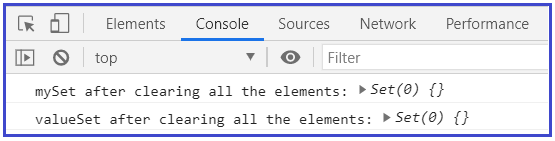##### Getting Set Length

Set give us a very straight-forward way to get back the size of our created Set. Simple use of the size property. We can get the number of elements or elements count inside the Set by using the .size property. This property, unlike Array.prototype.length, is read-only, which means that we can’t change its value by assigning some other value to it.

##### Example1: JavaScript Set size example
```<html>
<title>JavaScript Set size example1</title>
<body>
<script>
var mySet = new Set();
console.log("Size of mySet is:", mySet.size);

var valueSet = new Set([1, 3, 3, 7]);
valueSet.size = 10 //size property is read-only. Hence can’t change it by assigning something to it.
console.log("Size of valueSet is:", valueSet.size);
</script>
</body>
</html>
```

Output: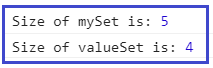In Strict mode, when we try to change the size property of Set it even throws an error: TypeError: Cannot set property size of #<Set> which has only a getter

##### Example2: Set size with strict mode
```<html>
<title>JavaScript Set size with strict mode example2</title>
<body>
<script>
"use strict"

var mySet = new Set();
console.log("Size of mySet is:", mySet.size);

var valueSet = new Set([1, 3, 3, 7]);
valueSet.size = 10 //size property is read-only. Hence can’t change it by assigning something to it.
console.log("Size of valueSet is:", valueSet.size);
</script>
</body>
</html>
```

Output: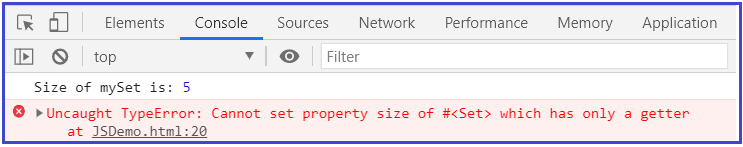##### Converting Sets to arrays

Sometimes we may need to convert a set to an array, when we want to filter the values of Set, for example, to be able to use Array.prototype methods like .filter(). In order to do so, we can use Array.from(). We can create an Array from a Set using Array.from or the spread operator. About Spread operator we will learn more in detail in their respective chapters. Set objects store unique values, so any duplicate elements from an Array are deleted when converting.

var mySet = new Set([11, 22, 33, 44]);
//use Array.from
const myArray = Array.from(mySet);
const myArray = […mySet];

Now we can filter the array to contain only even numbers and convert it back to Set using Set constructor:
mySet = new Set(myArray.filter(x => x % 2 === 0));

mySet now contains only even numbers:
console.log(mySet); // Set {2, 4}

##### Example1: using Array.from
```<html>
<title>JavaScript Converting Sets to arrays using Array.from example1</title>
<body>
<script>
var mySet = new Set([1, 2, 3, 4, 4, 5, 6, 7, 8, 9,]);

//use Array.from
const myArray = Array.from(mySet);
const destrMyArray = [...mySet];
console.log("mySet elements:", myArray);

mySet = new Set(myArray.filter(x => x % 2 === 0));
mySet1 = new Set(destrMyArray.filter(x => x % 2 === 0));
console.log("Converting Sets to arrays, using Array.from:", mySet); // Set {2, 4, 6, 8}
console.log("Converting Sets to arrays, using Spread operator:", mySet1); // Set {2, 4, 6, 8}
</script>
</body>
</html>
```

Output: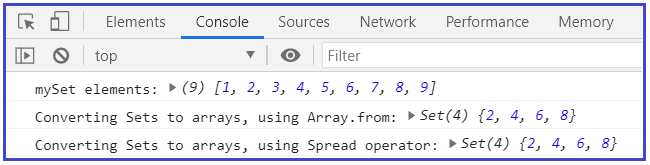##### Example2: using Array.from and Spread operator
```<html>
<title>JavaScript Converting Sets to arrays using Array.from and Spread operator example2</title>
<body>
<script>
var valueSet = new Set([1, 2, 3, 3, true]);
console.log("ValueSet:", valueSet);

//use Array.from
const myArray = Array.from(valueSet);
console.log("Converting Sets to arrays, using Array.from:", myArray);
</script>
</body>
</html>
```

Output: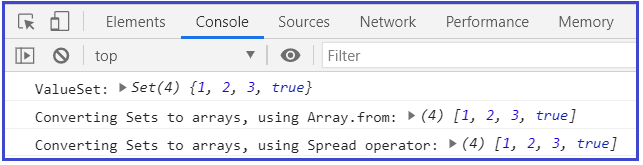##### Example3: Converting array to Set and then converting Set to Array using spread operator
```<html>
<title>JavaScript Relation with Array Objects-Converting array to Set and then converting Set to Array using spread operator example</title>
<body>
<script>
let myArray = ['array', 'set', 'spread']
console.log("original myArray", myArray);

// Use the regular Set constructor to transform an Array into a Set
let mySet = new Set(myArray)

mySet.has('array');     // returns true
console.log("Is mySet have 'array' element?:", mySet.has('array'));

// Use the spread operator to transform a set into an Array.
console.log("returning the same Array as myArray", [...mySet]) // Will show us exactly the same Array as myArray
</script>
</body>
</html>
```

Output: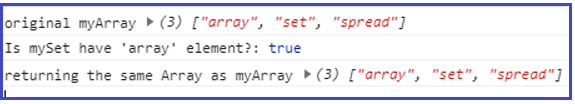##### Example4: Remove duplicate elements from the array
```<html>
<title>JavaScript Set to Remove duplicate elements from the array example</title>
<body>
<script>
// Use to remove duplicate elements from the array
const numArray = [2, 3, 4, 5, 2, 7, 3, 4, 4, 5, 5, 6, 6, 7, 5, 22, 8, 4, 5, 10, 10]
console.log("Original numArray: ", numArray)

console.log("The numArray after removing duplicate element: ",[...new Set(numArray)])
</script>
</body>
</html>
```

Output: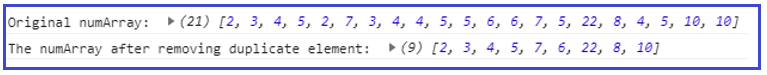##### Example5: Set relation with Strings with case sensitive and duplicate deletion
```<html>
<title>JavaScript Set relation with Strings with case sensitive and duplicate deletion example</title>
<body>
<script>
let city = 'Delhi'

let mySet = new Set(city)  // Set ['D', 'e', 'l', 'h','i']
console.log("original mySet when string value stored: ", mySet);

mySet.size  // 5
console.log("Size of mySet: ", mySet.size);

//case sensitive & duplicate ommision
let mySet1 = new Set("Mumbai")  // Set(6) [ "M", "u", "m", "b", "a", "i" ]
console.log("mySet1 with case sensitive value & no duplicate deletion: ", mySet1);

let mySet2 = new Set("mumbai")  // Set(6) [ "m", "u", "b", "a", "i" ]
console.log("mySet2 with non-case sensitive value & duplicate deletion: ", mySet2);
</script>
</body>
</html>
```

Output: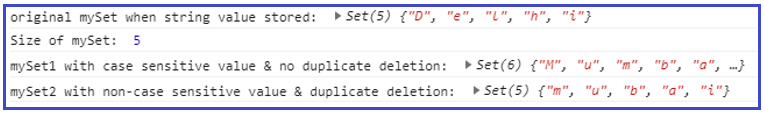##### Intersection and differences in Sets

There are no built-in methods for intersection and difference in Sets, but we can still achieve that by converting them to arrays, then filtering, and then converting back to Sets:

##### Example: JavaScript Sets Intersection and differences example
```<html>
<title>JavaScript Sets Intersection and differences example</title>
<body>
<script>
var valueSet1 = new Set([1, 2, 3, 4]),
valueSet2 = new Set([3, 4, 5, 6]);

//use Array.from
const intersection1 = new Set(Array.from(valueSet1).filter(x => valueSet2.has(x)));//Set {3, 4}
const difference1 = new Set(Array.from(valueSet1).filter(x => !valueSet2.has(x))); //Set {1, 2}

const intersection2 = new Set([...valueSet1].filter(x => valueSet2.has(x)));//Set {3, 4}
const difference2 = new Set([...valueSet1].filter(x => !valueSet2.has(x))); //Set {1, 2}

console.log("Sets Intersection--Array.from :", intersection1);
console.log("Sets Differences--Array.from:", difference1);
</script>
</body>
</html>
```

Output: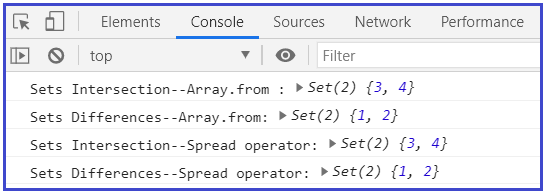##### Iterating Sets in JavaScript

We can use a simple for-of loop to iterate a Set.

const mySet = new Set([1, 2, 3]);
for (const value of mySet) {
console.log(value); // logs 1, 2 and 3
}

When iterating over a Set, it will always return values in the order they were first added to the Set.

For example:
const set = new Set([4, 5, 6])
Array.from(set) //[4, 5, 6, 10]

There’s also a .forEach() method, same as Array.prototype.forEach(). If we want to invoke a callback function on each element of a set, we can use the forEach() method.

forEach(callback [, thisArg])

It has two parameters, first is callback, which will be executed once for each element present in the Set object in the insertion order, and Second is optional thisArg. If thisArg  parameter is provided then it will be used as the this value for each call of callback function.

callback has three parameters. The first two parameters are both the current element of Set (for consistency with Array.prototype.forEach() and Map.prototype.forEach()) and the third argument is the Set itself.

In other words, callback function has 3 parameters. a value then the same value sameValueAgain and then the target object (like Set or arrays or map).

mySet.forEach((value, valueAgain, set) => console.log(value)); / logs 1, 2, 3, 4 and 5

##### Example: JavaScript Iterating Sets example
```<html>
<title>JavaScript Iterating Sets example</title>
<body>
<script>
const mySet = new Set([4, 5, 6])
Array.from(mySet) //[4, 5, 6, 10]
console.log("Convert Sets to array:", Array.from(mySet))

//Iterate mySet entries with for-of loop
for (const item of mySet) {
console.log("Iterate mySet entries with for-of loop", item);
}

//Iterate mySet entries with forEach()
mySet.forEach(function (value) {
console.log("Iterate mySet entries with forEach()", value);
});
</script>
</body>
</html>
```

Output: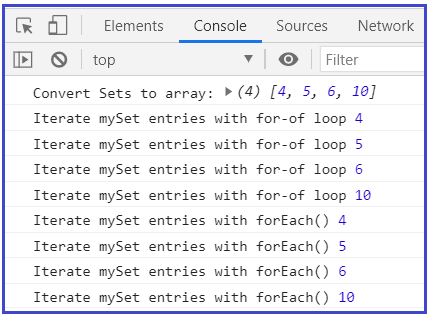##### entries(), keys(), and values() method of Set

The entries() method, returns an object of Set Iterator that contains an array of [value, value] for each element. The Set also provides the keys(), values(), and entries() methods like the Map. However, keys and values in the Set are identical.

##### Example: JavaScript Iterating Sets entries() method example
```<html>
<title>JavaScript Iterating Sets entries() method example</title>
<body>
<script>
const mySet = new Set([4, 5, 6])
Array.from(mySet) //[4, 5, 6, 10]
console.log("Convert Sets to array:", Array.from(mySet))

//Iterate mySet entries with for-of loop
for (let [key, value] of mySet.entries()) {
console.log("Is keys and values in the set are identical with for-of loop:keys:", key, "values:", value, "identical?", key === value);
}

</script>
</body>
</html>
```

Output: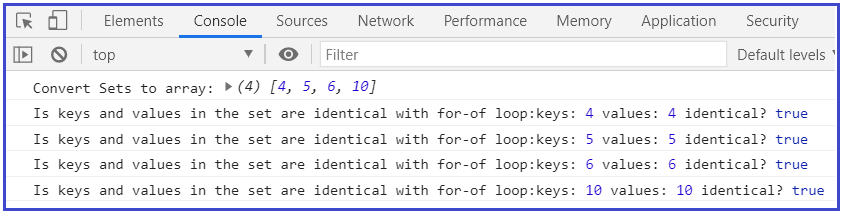##### Array Vs Set in JavaScript
• The array is defined with square brackets [ ] whereas Set is defined with curly braces { }.
• An array can contain duplicate values but in Set, it contains Unique values no duplicate.
• The array is an Indexed Collection type of data structure as in this each item is ordered by an index value, while Set is keyed Collection type of data structure as this uses keys, which contains items that are inserted in the order items are inserted.
• In Array new element is added by using the push() method while in the Set new element is added by using the set.add() method.
• In Array element is removed by using the pop() method while in Set it is removed by using the set.delete() method.
• To check whether an element exists in a collection using indexOf for arrays is slow.
• Set objects allow us to delete elements by their value. With an array, we would have to splice based on an element’s index. (arr.splice(arr.indexOf(val), 1))
• The value NAN cannot be found with indexOf in the array
• Set Objects store unique values, we don’t have to keep track of duplicates by ourself.
• Set for storing unique value collections whereas Array for storing ordered